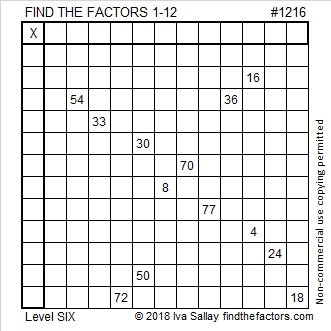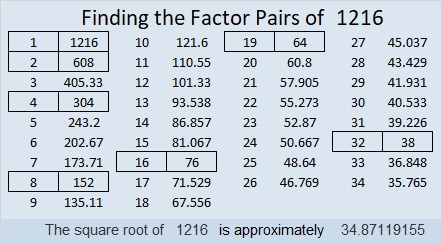# 1216 and Level 6

Is 6 or 9 the needed common factor for 54 and 36?
The other one will be the common factor for 72 and 18.

Will 5 or 10 be the common factor of 30 and 50?
Will 2 or 4 be the common factor of 16 and 4?

Don’t guess the answers to those questions! The other clues in the puzzle will help you find the answers. Will you be stumped or will you triumph?Print the puzzles or type the solution in this excel file: 12 factors 1211-1220

Now I’ll share some information about the number 1216:

• 1216 is a composite number.
• Prime factorization: 1216 = 2 × 2 × 2 × 2 × 2 × 2 × 19, which can be written 1216 = 2⁶ × 19
• The exponents in the prime factorization are 6, and 1. Adding one to each and multiplying we get (6 + 1)(1 + 1) = 7 × 2 = 14. Therefore 1216 has exactly 14 factors.
• Factors of 1216: 1, 2, 4, 8, 16, 19, 32, 38, 64, 76, 152, 304, 608, 1216
• Factor pairs: 1216 = 1 × 1216, 2 × 608, 4 × 304, 8 × 152, 16 × 76, 19 × 64, or 32 × 38
• Taking the factor pair with the largest square number factor, we get √1216 = (√64)(√19) = 8√19 ≈ 34.871191216 is the sum of the ten prime numbers between 100 and 150:
101 + 103 + 107 + 109 + 113 + 127 + 131 + 137 + 139 + 149 = 1216

1216 is the 19th nonagonal number because
19(7·19 – 5)/2 = 1216, in other words because 19 × 64 = 1216

This site uses Akismet to reduce spam. Learn how your comment data is processed.